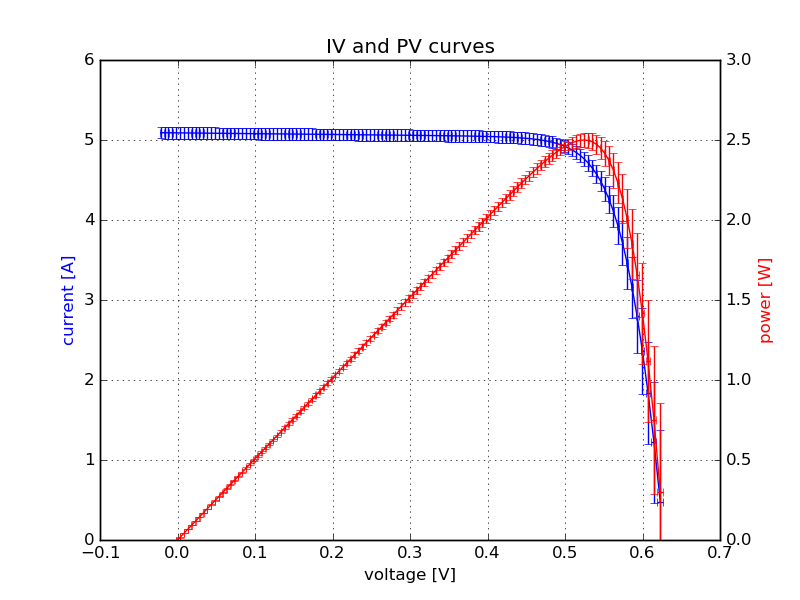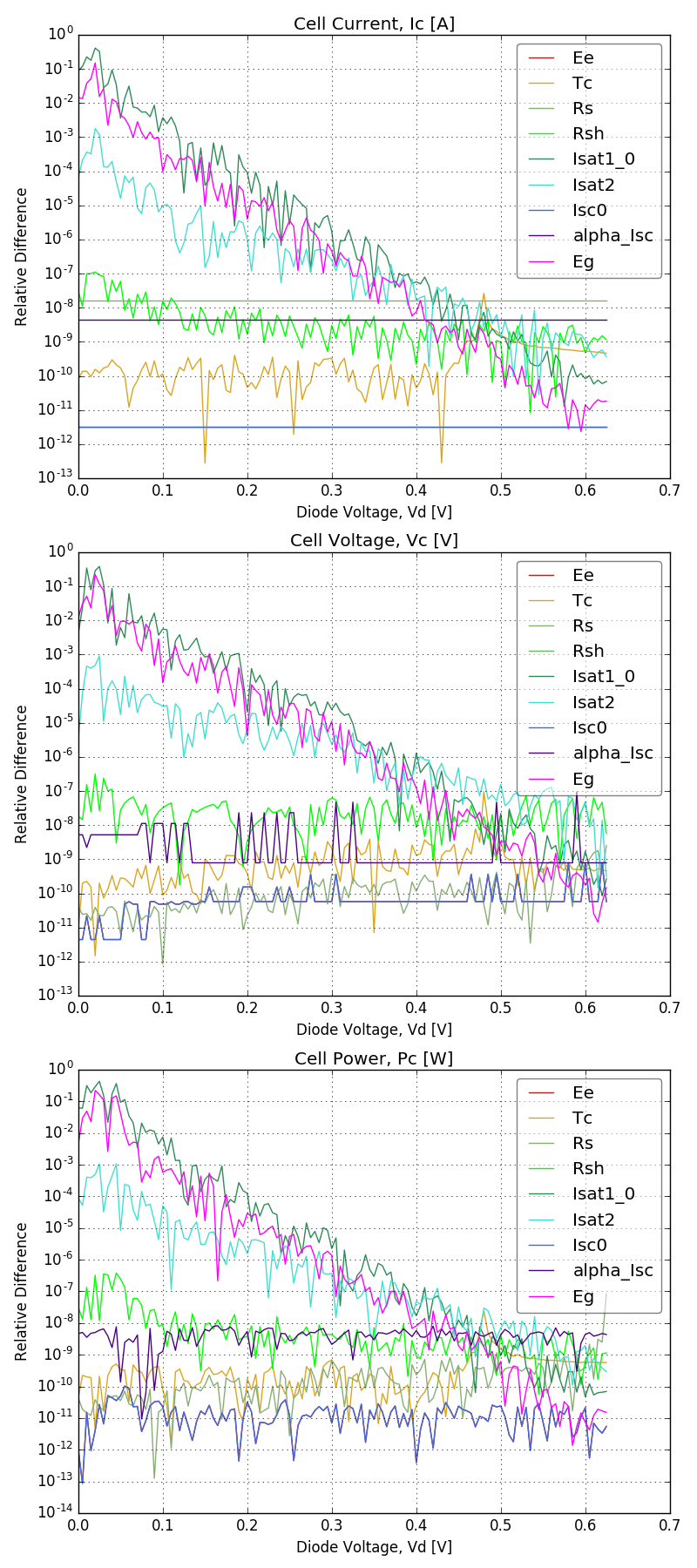# Complex Example¶

A more complex example from the test_uncertainty_wrapper module called test_IV(), includes combinations of several exponential and power operations. It contains 9 input arguments, there 126 observations of each corresponding to different voltages and there are 3 return values. The calculated uncertainty using a 1% standard deviation (square root of variance) for all 9 inputs is shown below.The test compares the derivatives calculated using central finite difference approximation with an analytical calculation from 0.3[V] to 0.6[V]. Below 0.3[V] the approximations deviate from the analytical for $$\frac{\partial I_{sc}}{\partial I_{sat_{1,0}}}$$, $$\frac{\partial I_{sc}}{\partial I_{sat_2}}$$ and $$\frac{\partial I_{sc}}{\partial E_g}$$ while all other independent variables are consistently below 10e-7. The analytical derivatives are propagated using AlgoPy, an automatic differentiation package, which requires rewriting all NumPy operations like $$exp$$ using AlgoPy. This makes it impractical for use in most models, but still useful for testing.